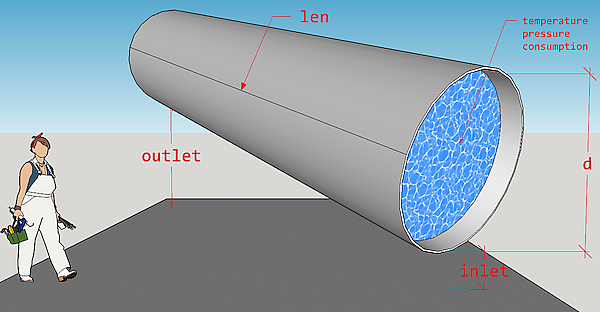Calculate pressure drop in straight circular steel pipe of district heating system (where water is a heat carrier) that is a result of pipe orientation in space (hydrostatic component), and friction between water and internal wall of pipe.

dropp(
temperature = 130,
pressure = mpa_kgf(6),
consumption = 1276,
d = 1,
len = 1,
roughness = 0.006,
inlet = 0,
outlet = 0,
method = "romeo"
)

## Arguments

temperature

temperature of heat carrier (water) inside the pipe, [°C]. Type: assert_double.

pressure

absolute pressure of heat carrier (water) measured at the entrance (inlet) of pipe, [MPa]. Type: assert_double.

consumption

amount of heat carrier (water) that is transferred by pipe during a period, [ton/hour]. Type: assert_double.

d

internal diameter of pipe, [m]. Type: assert_double.

len

pipe length, [m]. Type: assert_double.

roughness

roughness of internal wall of pipe, [m]. Type: assert_double.

inlet

elevation of pipe inlet, [m]. Type: assert_double.

outlet

elevation of pipe outlet, [m]. Type: assert_double.

method

method of determining Darcy friction factor. Type: assert_choice. (see Details)

## Value

pressure drop at the outlet of pipe, [MPa]. Type: assert_double.

## Details

The underlying engineering model for calculation of pressure drop considers only two contributions (components):

1. Pressure drop due to gravity (hydrostatic component).

2. Pressure drop due to friction.

The model does not consider any size changes of pipe and presence of fittings.

For the first component that depends on pipe position in space the next figure illustrates adopted disposition of pipe.So, the expression for the first component can be written as:

$$g \rho (outlet - inlet)$$

where g - is gravity factor, $$m/s^2$$, and $$\rho$$ - density of water (heat carrier), $$kg/m^3$$; inlet and outlet are appropriate pipe elevations (under sea or any other adopted level), $$m$$.

The second component comes from Darcy–Weisbach equation and is calculated using heating carrier regime parameters (temperature, pressure, consumption). Temperature and pressure values of heat carrier define water properties according to IAPWS formulation.

Several methods for calculating of Darcy friction factor are possible and limited to the next direct approximations of Colebrook equation:

romeo

Romeo, Royo and Monzon, 2002

vatankhan

buzelli

Buzzelli, 2008

According to Brkic, 2011 approximations errors of those methods do not exceed 0.15 % for the most combinations of Reynolds numbers and actual values of internal wall roughness of pipe.

dropt for calculating temperature drop in pipe

Other district heating: dropg(), dropt()

## Examples

# Typical pressure drop for horizontal pipeline segments
# in high-way heating network in Novosibirsk
dropp(len = c(200, 300))
#>  0.0007000666 0.0010500999

# 0.0007000666 0.0010500999